## 7.08.2012

### Trigonometric functions determination through a triangle

Trigonometric functions can be determined on a rectangular triangle. On a picture you can see determinations of all trigonometric functions through the elements of rectangular triangle.It is another icon for hanging out on a wall and overlearnings of text. We this we will not engage in. We will look better, as possible to combine a rectangular triangle and circumference. For this purpose we will combine two pictures: circumference from classic determination of trigonometric functions and our triangle. We will place a triangle next to a circumference. That is it looks.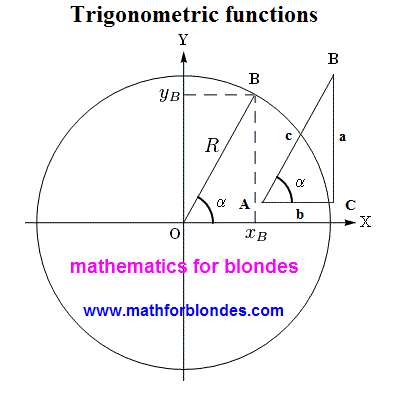As see, pictures are practically identical, and component elements are named differently in them. Identically mark only corner alpha and point of "B". Now we will impose a triangle straight on a circumference. All graphic images accepted for a circumference we will save, and a rectangular triangle we will underline red lines on the inside (type we will lead around the contour of triangle lipstick).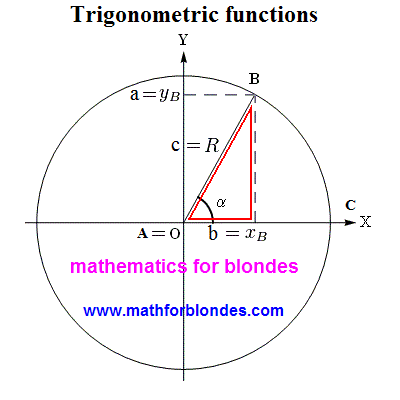As be obvious from a picture, the hypotenuse of rectangular triangle grows into the radius of circumference, cathetuses become equal to the coordinates of point. It as in a human language - a the same concept in different languages is designated by different words. This distinction in a pronunciation does not give to us to understand foreign languages. Approximately the same takes place in mathematics. Some consider determination of trigonometric functions on a rectangular triangle primitive. If you want to understand mathematics, will memorize the following: there are not primitive things in mathematics. There are primitive creatures counting itself very clever. Exactly desire to seem more clever than other, resulted in that mathematicians almost nothing is understood in mathematics.

Specially I will quote a phrase from Russian-language Wikipedia, where talked about determination of trigonometric functions on a triangle: "This determination has some pedagogical advantage, because does not require introductions of concept of the system of coordinates, but also such large defect, that it is impossible to define trigonometric functions even for obtuse angles that must be known at the decision of elementary tasks about amblygons". Honestly speaking, like thick ignorance of mathematicians simply shocks me. What triangle are trigonometric functions determined for? Correctly, for RECTANGULAR. Let though one mathematician will show to me RECTANGULAR TRIANGLE With OBTUSE ANGLE. I swear to you, as soon as I will see this geometrical miracle, I here will invert the portrait of tangent heels over head and I will hang oneself on a cosine. Not a single mathematician understands really, that the decision of "elementary tasks about amblygons" through trigonometric functions is ALWAYS taken to breaking up of one amblygon on two rectangular triangles?

Now I will show the most primitive example of existence of trigonometry you in life. More primitive, probably, is not already.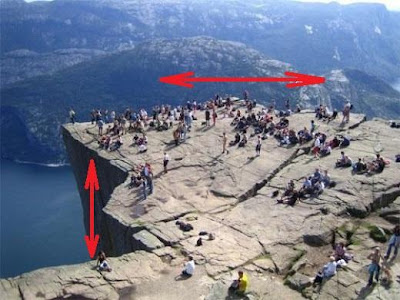Look at a photo - how many human bodies serenely stationed oneself on the horizontal plane of rock, at the same time there is nobody on a vertical plane. Why? And because trigonometric functions for perpendicular direction have quite another values. For us with you basic sense of trigonometric functions is that exactly trigonometric functions determine our possibilities.

## 7.03.2012

### Trigonometric functions are determination

Determination of trigonometric functions I took from Russian-language part of Wikipedia and designed as a picture. You can look and read.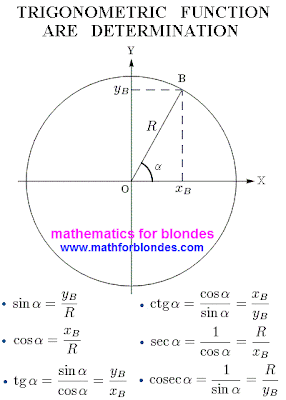Picture, naturally, I a bit did. In Wikipedia text of determination of trigonometric functions is written about one - about the coordinates of point and radius of circumference, and on a picture quite another is drawn. Probably, an artist from Wikipedia looked not in the that page of textbook on mathematics. Well yet, that he the textbook of mathematics with a textbook on biology did not entangle. Here that turned out for him.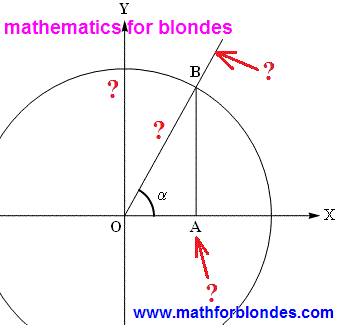Many of you can say: "I do not understand trigonometry. That to do"? Do the same, that do all mathematicians - bluntly teach determinations and, if necessary, pronounce them aloud, as talking parrots. For this purpose unseal my picture, hang up it above a bed and every evening before a dream read determination of trigonometric functions instead of prayer. I will open to you one great secret of mathematicians : those, who rewrites the determination given above from a textbook in a textbook, very small understand in mathematics in general, and quite nothing is understood in trigonometric functions in particular. Those, who understands, what trigonometric functions and able to use them, on all Universe travel in flying piattis. We hardly tear away the priests from a terrene through airplanes and rockets. By the way, would you like to exchange it stinking petrol car on an elegant flying object of UFO type?

If did you already read my notes How to memorize trigonometric functions? and the Trigonometric functions, then to you teaching nothing is not necessary. All, that it is needed for formulation of any determination of trigonometric functions, you know already. Later I will show you, as to use these knowledge. And while we will keep reading Wikipedia, where talked about determination of trigonometric functions for a rectangular triangle.

## 7.02.2012

### Trigonometric functions

Trigonometric functions is simply. At the last time we considered the portrait of tangent, today we will expose the secrets of practical application of this masterpiece of painting.

Any trigonometric function is the result of division. It needs to be memorized forever. We divide something by something and as a result get a trigonometric function. That on what divided in trigonometric functions? Variants are different here. I suggest not to learn by rote you on memory all, in respect of trigonometric functions. Let us try simply to apply the portrait of tangent and minimum set of necessary knowledge.

And so, a tangent is a sine on a cosine. As we said hardly higher, in a tangent a sine is divided by a cosine. A division in mathematics can mark a fractional line or two points. We will transform our portrait of tangent in the record of partaking with two points. Here that turned out for us.Most important, what we are taught by this picture, so it to those places, where it is needed to search sines and cosines. In the system of denotations accepted by mathematicians, sines always are situated on a vertical line - it upwards-downward. Cosines always are situated on a horizontal - it to the left-right (or aside). That is a complete set will look from 6 trigonometric functions in our variant of denotations. For evidentness a sine and cosine are depicted by a different color and we will add fractional hyphens wherein without them not to do.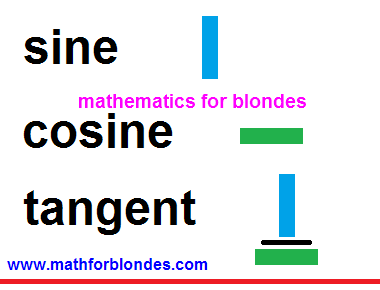Do not be frightened such amount of pictures. That it is represented in overhead part, above a red line, we almost learned already. We will repeat:

## a sine - it upwardsa cosine - is asidea tangent - it to divide a sine into a cosine

Do you see? The half of trigonometric functions we learned already. With the second half, that under a red line, yet simpler - invert that you learned already heels over head. Remaining trigonometric functions are shots reverse already known to us. We swap a numerator and denominator - all is ready already for us.

With a cotangent all is simple. A cotangent is this attitude of cosine toward a sine. With gimbleting of tangent no problems arise up - he has both a numerator and denominator. But as to be with a sine and cosine? We do not have denominators of these shots in fact! Do not worry, any number has a denominator that in mathematics, writing is not accepted is unit. In a secant and cosecant exactly this unit appears in a numerator, and a cosine and sine are written in a denominator.

It is important to memorize! In the pairs of the names of trigonometric functions a secant is a cosine and cosecant - a sine can be only one prefix to on two names. If we take a sine for basis, then a reverse shot will be named cosecant. If we take a cosine, then a reverse shot is named secant, because one prefix to we already used up on a cosine.

It is important to memorize! In the pairs of the names of trigonometric functions a secant - is a cosine and cosecant - a sine can be only one prefix co on two names. If we take a sine for basis, then a reverse shot will be named cosecant. If we take a cosine, then a reverse shot is named secant, because one prefix co we already used up on a cosine.

In principle, it fully sufficiently, in determinations of trigonometric functions to feel, close as an oyster in water. A question remains not found out, that at a sine and cosine to write in a denominator instead of unit. In fact we said in beginning, that trigonometric functions are a result of division(relation) or shot. Do not worry, mathematicians will prompt us, that exactly they want there to see. For this purpose there are determinations of trigonometric functions. We will talk next time about them.

### Why is a zero not a number?

Why is a zero not a number? Let us conduct a scientific experiment. We will take any number and will execute the simplest mathematical action - addition or deduction.

Simply so to execute a mathematical action will not turn out. One number and one sign of mathematical action are simply two mathematical symbols, standing alongside. Single character designates a number, the second symbol designates an action.

7 +
7 –

In order that an action happened, it is needed yet something. Only then we will get a result. And this result depends on that we take exactly. If we will take other any number, then the first number will change. The result of this change we write down after equal sign.

7 + 2 = 9
7 – 2 = 5

number + number = result
number - number = result

And that will be, if we will take a not number? For example, will we add salt, will add a paint, will decrease a temperature? That will be with a number, if to salt him? Nothing. A number will remain unchanging.

7 + salt = 7

Now we will try to paint a number.

7 + paint = 7

A number did not change again. But as regarding that, to push in our number in a refrigerator and lower his temperature?

7 - temperature = 7

Why does take place so? Because salt, paint, temperature numbers it is not been. A number and not number inter se do not co-operate. If to take them and make an effort with them to do anything, for us nothing will turn out. What number we took at the beginning, the same we get in the end.

number + not number = number and not number
number - not number = number and not number

Mathematicians and philosophers will argue that a number is an abstract concept that does not exist in the wild, and salt, paint, temperature, is the real things. Exactly from a difference abstract and real nothing takes place. The abstract can not influence on the real, real can not influence on abstract. What, I fully agree with the conclusions of mathematicians and philosophers. They are absolutely right - for a change real the real is needed, for a change abstract the abstract is needed.

In the second part of our experiment we will try to the abstract number to add anything abstract. Well, for example, soul. In hands this thing nobody held, she is not fixed scientific devices. The soul is this cleanly abstract concept. We will look at a result.

7 + the soul = 7
7 - the soul = 7

As see, even many abstract concepts are not able to run the number, because not all abstract concepts are numbers.

Here time to conduct an experiment with a zero, the same abstract concept, as well as number came now. We will add and will take away a zero from a number and will look at a result.

7 + 0 = 7
7 – 0 = 7

As a result of addition or deduction of zero a number remains unchanging. And takes place so because zero, as well as all other not numbers, a number is not. A zero does not possess all those properties that is possessed by numbers.

For plenitude of the conducted experiment it is needed to consider the mathematical operations of increase and division. Multiplying and dividing by a zero in mathematics has a result different from the originally taken number. But let us remember history of development of mathematics.

Addition and deduction appeared at first, an increase and division appeared then. Probably, somewhere a zero appeared after it. There was it in those times, when a faith in God was basis of existence of man. About no results of experiments there could not be speech, if these results conflicted with a faith. Here on this faith and the results of increase and dividing are founded by a zero. Someone said that a zero was a number and in it believed. Someone offered the result of multiplying by a zero - believed in a result. In him we are pin faiths until now: any number increased on a zero equals a zero.

With dividing by a zero there were problems. Nobody was able to offer nothing clever, such, that all believed in it. Mathematical experiments with the results of dividing by a zero give contradictory results and trench upon bases of mathematics. A compromise variant was therefore found is a phrase, "dividing by a zero is impossible". It did not mix to believe that a zero is a number, and did not result in the necessity of revision of other substantive provisions of mathematics. Absence of result of division of number on a zero confirms circumstance that in this case we deal with a not number.

Conclusion: ZERO IS NOT NUMBER

## 2.28.2012

### Modulus of sine

Me it was asked to show method to simplify trigonometric expression, containing the sum of sine and modulus of sine of corner, knowing that corner alpha is finished in 4 fourths. This expression looks so:

|sinA|+sinA

At once I will say honestly, that I a concept do not have, as such expressions are simplified. But about the module of sine I can tell and that will turn out in the total, then. All of you know well, that a sine, as well as all trigonometric functions, can take on positive and negative values. So, sine in the Chinese sticks, that in mathematics read as a "module of sine of corner A", can not have negative values, only positive. When mathematicians are fastidious to touch to the negative numbers, they apply these chopsticks (or modulus of number), as condom at sex, that to be not infected by minus. They rescue the life these, as all numbers in the module from negative grow into positive.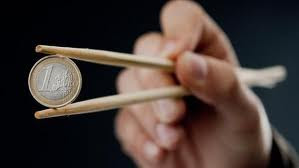Well now a bit about sign life of sine of corner of А. Sine - it for us upwards and downward on an axis Y-mill from unit to minus units. When corner A from 0 to 180 degrees take on values, all sines of these corners are positive. In this case the chopsticks of the module are the superfluous measure of caution and they can be cast aside. In this range of values of corner A our expression will assume an air:

|sinA|+sinA = sinA+sinA = 2sinA (0 < A < 180)

If value of corner A to increase farther, from 180 to 360 degrees, values of sines of these corners will be negative, id est with a sign " minus". In this case the module begins to play the rock role the fate of our mathematical expression. The value of sine with the module remains positive, and the value of sine without the module becomes negative, as well as it is fixed to all decent sines. What will we get, if from a number we will take away a such is exact number? Correctly, zero. Our expression dies out, as dinosaurs. By the way, if all people always will use condoms during sex, humanity here will die out fully. Effect of the modulus. We will look, that takes place with our expression in this case:

|sinA|+sinA = sinA-sinA = 0 (180 < A < 360)

Application of formulas of bringing trigonometric functions over will give an exactly such result. Thus the module compels us to cast aside in garbage all signs minus, got as a result of transformations.

At corners 0, 180, 360 et cetera degrees our expression will equal a zero, as a zero the values of sine of these corners are equal to.

As all of it it is correct to write down in complete accordance with the rules of mathematical bureaucracy, I do not know. But sense of what be going on, I hope, clear you and you without effort will design this expression in the best kind.

## 2.14.2012

### Trigonometric table in radians

This trigonometric table is made for the values of corners in radians. Radians are here given as decimal fractions within two signs after a comma. Value of sine, cosine and tangent given within four signs after a comma. It is such small trigonometric table in radians.

## Table of trigonometric values in degrees:sin costan cot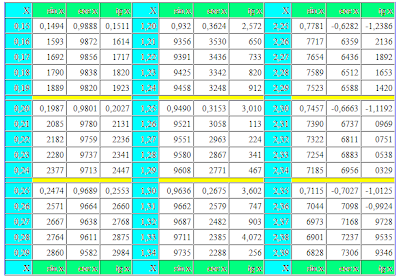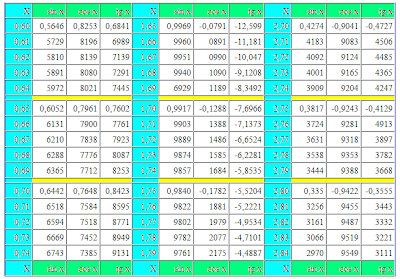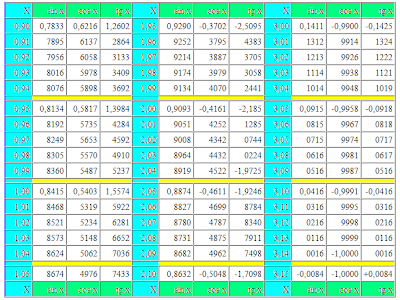In this trigonometric table the value of corner in radians closes on a 3,15 radian, that corresponds hardly anymore 180 degrees in the degree measure of corners. Here you will not find the value of tangent, equal to unit, value of sine, equal to unit and value of cosine, equal to the zero. In the radian measure of corners to get these values unassisted number of Pi it is impossible. And as a self number of Pi is an endless shot not having the exact meaning, expediency of goniometry in radians is very doubtful. Radians - it, put it mildly, strange unit of measurement.

Values of corner in radians are in blue columns mark the letter of "X". In three columns the values of sin x are given on the right, cos x and tg x for corners in radians. Value of cotangent, secant and cosecant to the table not driven, as these trigonometric functions are reverse shots to driven to the table. For the receipt of values of ctg x, sec x and cosec x in radians, it is needed to divide unit into a tangent, cosine or sine of corresponding corner in radians.

## 2.04.2012

### Trigonometric table tangent cotangent in degrees

Trigonometric table tangent cotangent in degrees - it is the four-valued table of tangents and cotangents in degrees within one minute. A few examples are made at the beginning, as to use this table. For comfort in a table additional navigation elements are plugged with pointing by the name of the names of functions and values of minutes column-wise for every function. Value of degrees and minutes for every trigonometric function distinguished by different colors: for tangents - green, for cotangents - blue. Connotations for minutes are distinguished by yellow.

A trigonometric table for tangents and cotangents consists of two parts. The first part includes tangents from 0 to 75 degrees and cotangents from 15 to 90 degrees. Before to use this table, it is recommended on present examples to find the values of tangents and cotangents on a table and compare results. If all turned out correctly for you, means you can consider itself an experience user of table.At the use it is necessary to remember this table, that additional values one, two and three minutes for a tangent have a sign plus and at addition added and at deduction subtracted, for a cotangent they have a sign minus and at addition subtracted, and at deduction added. For verification compare the got result to the values of the same name trigonometric functions in the alongside located cells.

The second part of trigonometric table includes tangents from 75 to 90 degrees and cotangents from 0 to 15 degrees. To use this table some simpler, than by the first part.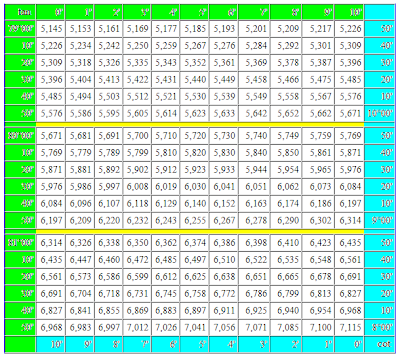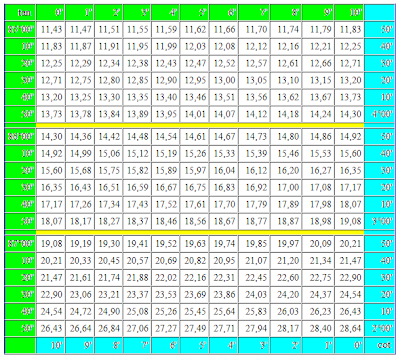For a tangent 90 degrees and cotangent 0 degrees a value is not certain. It is so accepted to consider because task on dividing by a zero mathematicians until now so not succeeded to decide.

If you need a trigonometric table of sines and cosines, then she can be found on a separate page.